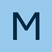# 2020 ICAS Assessments - Mathematics

UNSW Global

\$14.50

ICAS Mathematics

Skill Areas

Students are assessed across five key skill areas:

• Algebra and patterns: Patterns of numbers, relationships between numbers and the use of symbols to stand for unknown or variable numbers
• Chance and data: Mathematical treatment of data and statistics
• Measures and units: Properties of the physical world that can be measured, the units used to measure them and the process of measurement
• Number and arithmetic: Types of numbers, their properties and number operations. This strand has strong links with measures and units, algebra and patterns, and chance and data. It also has some links with space and geometry
• Space and geometry: Properties of two-dimensional and three-dimensional space.

Content

ICAS questions require students to make sense of mathematical concepts in everyday situations. Content differs across the papers to ensure they are appropriate for year levels.

Papers Introductory, A, B, C, D and E:

Formal algebra is not tested. The emphasis is put on pattern, structure and puzzles.

Formal geometry is tested only in Paper E. The emphasis is on spatial skills.

Papers F, G, H, I and J:

Formal algebra is tested. Students are expected to be familiar with some conventions of algebra.

Formal geometry is tested. Students are expected to be familiar with some conventions of geometry.